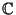## Field

A field is any set of elements which satisfies the Field Axioms for both addition and multiplication and is a commutative Division Algebra. An archaic name for a field is Rational Domain. A field with a finite number of members is known as a Finite Field or Galois Field.

Because the identity condition must be different for addition and multiplication, every field must have at least two elements. Examples include the Complex Numbers (), Rational Numbers (), and Real Numbers (), but not the Integers (), which form a Ring. It has been proven by Hilbertand Weierstraßthat all generalizations of the field concept to triplets of elements are equivalent to the field of Complex Numbers.

See also Adjunction, Algebraic Number Field, Coefficient Field, Cyclotomic Field, Field Axioms, Field Extension, Function Field, Galois Field, Mac Lane's Theorem, Module, Number Field, Quadratic Field, Ring, Skew Field, Vector Field